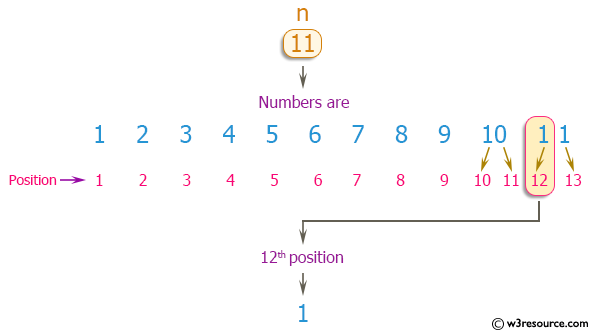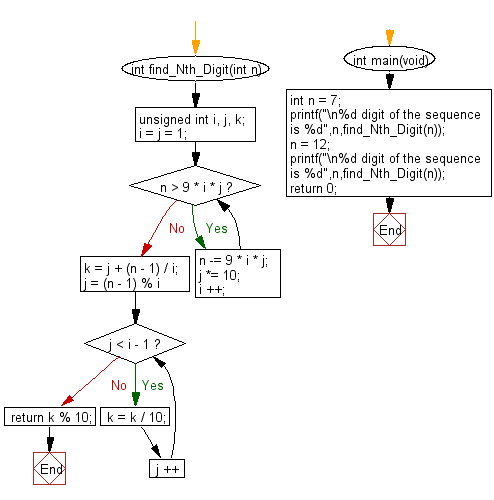﻿ C Program: Find the nth digit of number 1 to n - w3resource

# C Exercises: Find the nth digit of number 1 to n

## C Programming Mathematics: Exercise-17 with Solution

Write a C program to find the nth digit of the number 1 to n?
Infinite integer sequence: 1, 2, 3, 4, 5, 6, 7, 8, 9, 10, 11, 12 .. where n is a positive integer.

Example:
Input:
7
Output:
7
Input:
12
Output:
1
The 12th digit of the sequence 1, 2, 3, 4, 5, 6, 7, 8, 9, 10, 11, ... is 1, which is part of the number 11.

Pictorial Presentation:Sample Solution:

C Code:

``````#include <stdio.h>

int find_Nth_Digit(int n) {
unsigned int i, j, k;
i = j = 1;
while (n > 9 * i * j) {
n -= 9 * i * j;
j *= 10;
i ++;
}
k = j + (n - 1) / i;
for (j = (n - 1) % i; j < i - 1; j ++) {
k = k / 10;
}
return k % 10;
}

int main(void)
{
int n = 7;
printf("\n%d digit of the sequence is %d",n,find_Nth_Digit(n));
n = 12;
printf("\n%d digit of the sequence is %d",n,find_Nth_Digit(n));
return 0;
}
```
```

Sample Output:

```7 digit of the sequence is 7
12 digit of the sequence is 1
```

Flowchart:C Programming Code Editor:

Improve this sample solution and post your code through Disqus.

What is the difficulty level of this exercise?

Test your Programming skills with w3resource's quiz.

﻿